Скачать презентацию GUHA — a summary 1 GUHA General Unary

c715ed6bfbc6fc26c337e2c37db9abac.ppt

• Количество слайдов: 8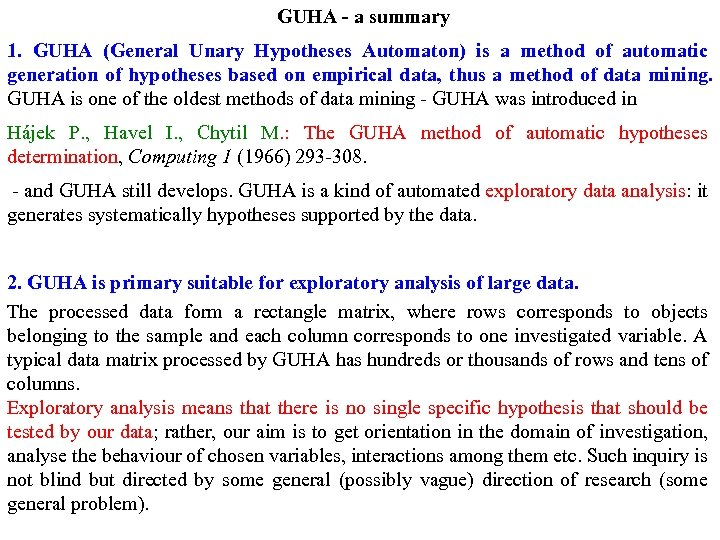GUHA - a summary 1. GUHA (General Unary Hypotheses Automaton) is a method of automatic generation of hypotheses based on empirical data, thus a method of data mining. GUHA is one of the oldest methods of data mining - GUHA was introduced in Hájek P. , Havel I. , Chytil M. : The GUHA method of automatic hypotheses determination, Computing 1 (1966) 293 -308. - and GUHA still develops. GUHA is a kind of automated exploratory data analysis: it generates systematically hypotheses supported by the data. 2. GUHA is primary suitable for exploratory analysis of large data. The processed data form a rectangle matrix, where rows corresponds to objects belonging to the sample and each column corresponds to one investigated variable. A typical data matrix processed by GUHA has hundreds or thousands of rows and tens of columns. Exploratory analysis means that there is no single specific hypothesis that should be tested by our data; rather, our aim is to get orientation in the domain of investigation, analyse the behaviour of chosen variables, interactions among them etc. Such inquiry is not blind but directed by some general (possibly vague) direction of research (some general problem).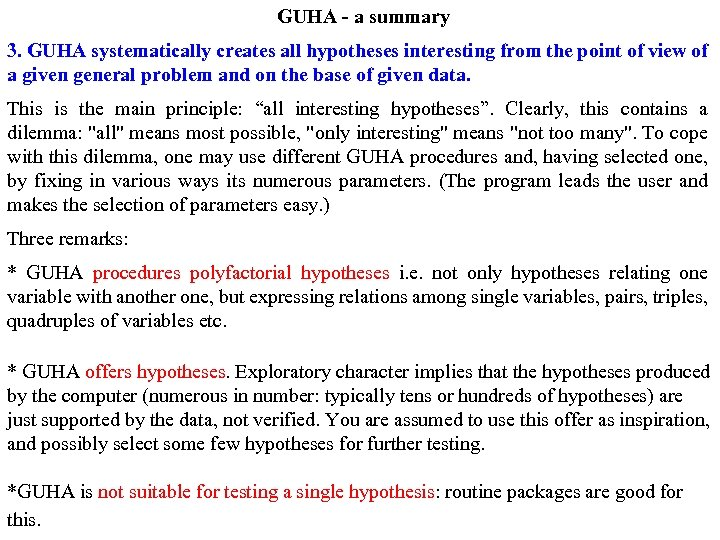GUHA - a summary 3. GUHA systematically creates all hypotheses interesting from the point of view of a given general problem and on the base of given data. This is the main principle: “all interesting hypotheses”. Clearly, this contains a dilemma: "all'' means most possible, "only interesting'' means "not too many''. To cope with this dilemma, one may use different GUHA procedures and, having selected one, by fixing in various ways its numerous parameters. (The program leads the user and makes the selection of parameters easy. ) Three remarks: * GUHA procedures polyfactorial hypotheses i. e. not only hypotheses relating one variable with another one, but expressing relations among single variables, pairs, triples, quadruples of variables etc. * GUHA offers hypotheses. Exploratory character implies that the hypotheses produced by the computer (numerous in number: typically tens or hundreds of hypotheses) are just supported by the data, not verified. You are assumed to use this offer as inspiration, and possibly select some few hypotheses for further testing. *GUHA is not suitable for testing a single hypothesis: routine packages are good for this.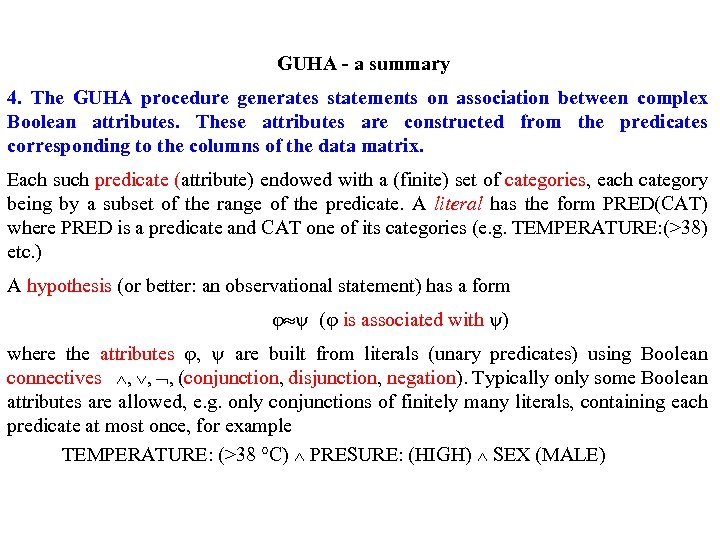GUHA - a summary 4. The GUHA procedure generates statements on association between complex Boolean attributes. These attributes are constructed from the predicates corresponding to the columns of the data matrix. Each such predicate (attribute) endowed with a (finite) set of categories, each category being by a subset of the range of the predicate. A literal has the form PRED(CAT) where PRED is a predicate and CAT one of its categories (e. g. TEMPERATURE: (>38) etc. ) A hypothesis (or better: an observational statement) has a form ( is associated with ) where the attributes , are built from literals (unary predicates) using Boolean connectives , , , (conjunction, disjunction, negation). Typically only some Boolean attributes are allowed, e. g. only conjunctions of finitely many literals, containing each predicate at most once, for example TEMPERATURE: (>38 °C) PRESURE: (HIGH) SEX (MALE)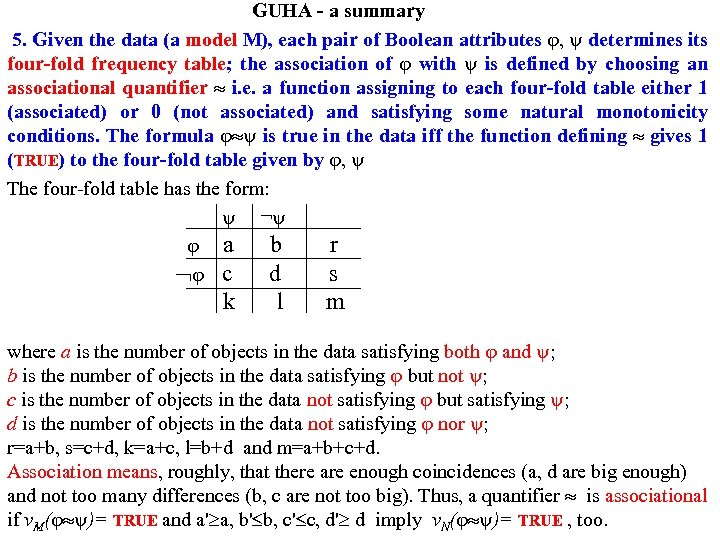GUHA - a summary 5. Given the data (a model M), each pair of Boolean attributes , determines its four-fold frequency table; the association of with is defined by choosing an associational quantifier i. e. a function assigning to each four-fold table either 1 (associated) or 0 (not associated) and satisfying some natural monotonicity conditions. The formula is true in the data iff the function defining gives 1 (TRUE) to the four-fold table given by , The four-fold table has the form: ¬ a b r c d s k l m where a is the number of objects in the data satisfying both and ; b is the number of objects in the data satisfying but not ; c is the number of objects in the data not satisfying but satisfying ; d is the number of objects in the data not satisfying nor ; r=a+b, s=c+d, k=a+c, l=b+d and m=a+b+c+d. Association means, roughly, that there are enough coincidences (a, d are big enough) and not too many differences (b, c are not too big). Thus, a quantifier is associational if v. M( )= TRUE and a' a, b' b, c' c, d' d imply v. N( )= TRUE , too.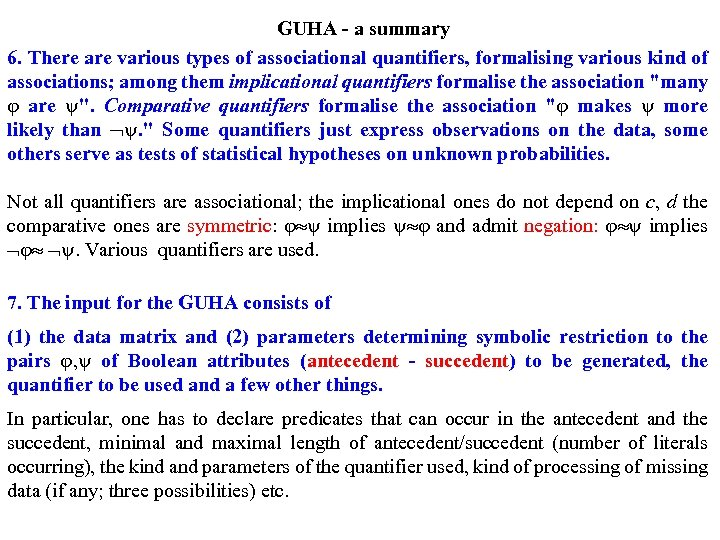GUHA - a summary 6. There are various types of associational quantifiers, formalising various kind of associations; among them implicational quantifiers formalise the association "many are ''. Comparative quantifiers formalise the association " makes more likely than . '' Some quantifiers just express observations on the data, some others serve as tests of statistical hypotheses on unknown probabilities. Not all quantifiers are associational; the implicational ones do not depend on c, d the comparative ones are symmetric: implies and admit negation: implies . Various quantifiers are used. 7. The input for the GUHA consists of (1) the data matrix and (2) parameters determining symbolic restriction to the pairs , of Boolean attributes (antecedent - succedent) to be generated, the quantifier to be used and a few other things. In particular, one has to declare predicates that can occur in the antecedent and the succedent, minimal and maximal length of antecedent/succedent (number of literals occurring), the kind and parameters of the quantifier used, kind of processing of missing data (if any; three possibilities) etc.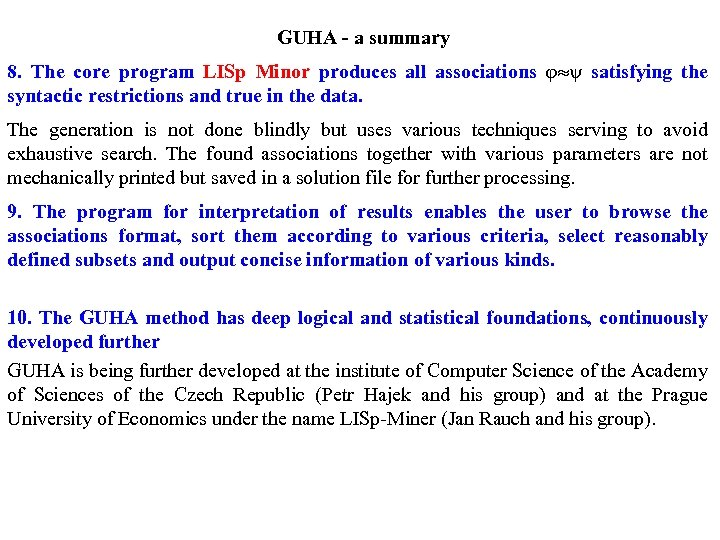GUHA - a summary 8. The core program LISp Minor produces all associations satisfying the syntactic restrictions and true in the data. The generation is not done blindly but uses various techniques serving to avoid exhaustive search. The found associations together with various parameters are not mechanically printed but saved in a solution file for further processing. 9. The program for interpretation of results enables the user to browse the associations format, sort them according to various criteria, select reasonably defined subsets and output concise information of various kinds. 10. The GUHA method has deep logical and statistical foundations, continuously developed further GUHA is being further developed at the institute of Computer Science of the Academy of Sciences of the Czech Republic (Petr Hajek and his group) and at the Prague University of Economics under the name LISp-Miner (Jan Rauch and his group).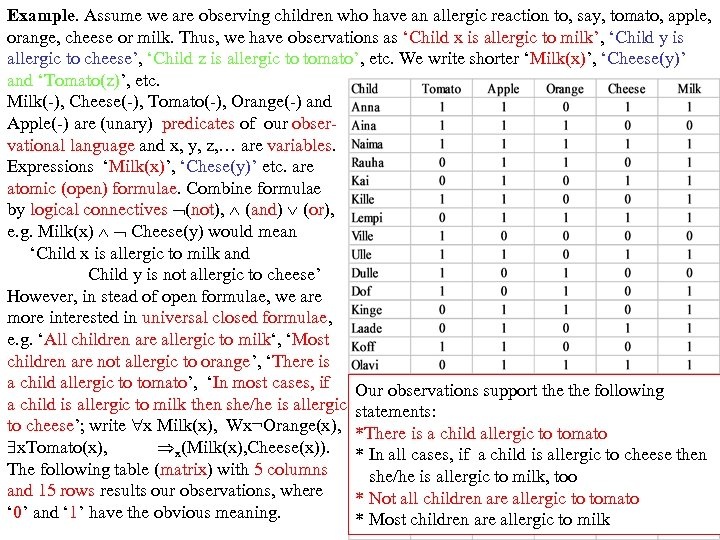Example. Assume we are observing children who have an allergic reaction to, say, tomato, apple, orange, cheese or milk. Thus, we have observations as ‘Child x is allergic to milk’, ‘Child y is allergic to cheese’, ‘Child z is allergic to tomato’, etc. We write shorter ‘Milk(x)’, ‘Cheese(y)’ and ‘Tomato(z)’, etc. Milk(-), Cheese(-), Tomato(-), Orange(-) and Apple(-) are (unary) predicates of our observational language and x, y, z, … are variables. Expressions ‘Milk(x)’, ‘Chese(y)’ etc. are atomic (open) formulae. Combine formulae by logical connectives (not), (and) (or), e. g. Milk(x) Cheese(y) would mean ‘Child x is allergic to milk and Child y is not allergic to cheese’ However, in stead of open formulae, we are more interested in universal closed formulae, e. g. ‘All children are allergic to milk‘, ‘Most children are not allergic to orange’, ‘There is a child allergic to tomato’, ‘In most cases, if Our observations support the following a child is allergic to milk then she/he is allergic statements: to cheese’; write x Milk(x), Wx¬Orange(x), *There is a child allergic to tomato x. Tomato(x), x(Milk(x), Cheese(x)). * In all cases, if a child is allergic to cheese then The following table (matrix) with 5 columns she/he is allergic to milk, too and 15 rows results our observations, where * Not all children are allergic to tomato ‘ 0’ and ‘ 1’ have the obvious meaning. * Most children are allergic to milk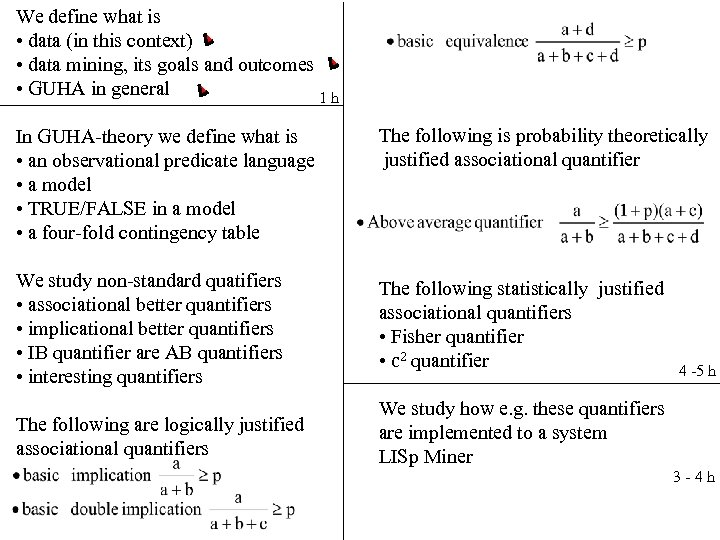We define what is • data (in this context) • data mining, its goals and outcomes • GUHA in general 1 h In GUHA-theory we define what is • an observational predicate language • a model • TRUE/FALSE in a model • a four-fold contingency table The following is probability theoretically justified associational quantifier We study non-standard quatifiers • associational better quantifiers • implicational better quantifiers • IB quantifier are AB quantifiers • interesting quantifiers The following statistically justified associational quantifiers • Fisher quantifier • c 2 quantifier The following are logically justified associational quantifiers We study how e. g. these quantifiers are implemented to a system LISp Miner 4 -5 h 3 -4 h